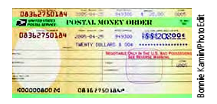Chapter 8.2, Problem 23ESMathematical Excursions (MindTap C...

4th Edition
Richard N. Aufmann + 3 others
ISBN: 9781305965584

Solutions

Chapter
SectionMathematical Excursions (MindTap C...

4th Edition
Richard N. Aufmann + 3 others
ISBN: 9781305965584
Textbook Problem

Money Orders Some money orders have serial numbers that consist of a 10-digit number followed by a check digit. The check digit is chosen to be the sum of the first 10 digits mod 9. Determine the check digit for the given money order serial number.0316615498-?

To determine

Money orders have special numbers that consists of a 10-digit number followed by a check digit. The check digit is chosen to be the sum of the first 10 digits mod 9. Determine the check digit for the given money order serial number as 0316615498?

Explanation

Given: Money order serial number as 0316615498?

Calculation:

Given the money order serial number as

0316615498?

Thus, the check digit is calculated as follows:

d11=(0+3+1+6+6+1+

Still sussing out bartleby?

Check out a sample textbook solution.

See a sample solution

The Solution to Your Study Problems

Bartleby provides explanations to thousands of textbook problems written by our experts, many with advanced degrees!

Get Started